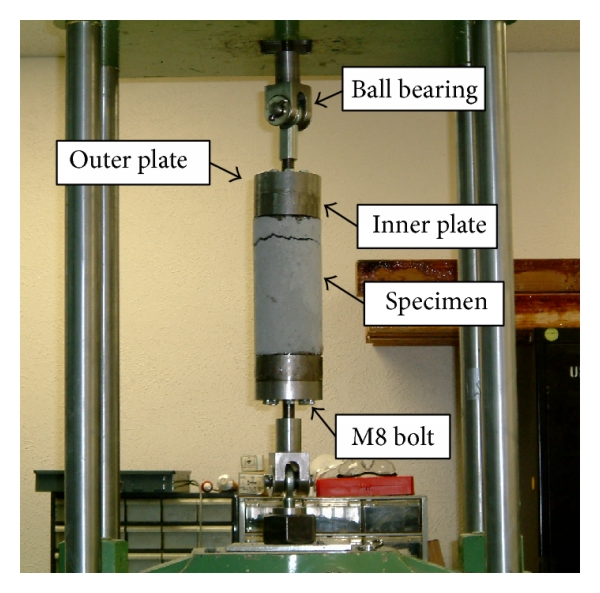### Methods of estimating concrete tensile strength

Concrete possesses a low tensile strength. The tensile strength of concrete estimated to be less than 10% of it is compressive strength. The low tensile strength of concrete explains the using of reinforcement bar in areas of tensile stress. Steel and concrete are compatible materials. Steel and concrete work in unison to resist both tensile and compressive stress. Various tests can be conducted to determine the tensile strength of concrete.

Split cylinder test used to estimate the tensile strength of concrete. Cylinder concrete specimen of 150 mm diameter and 300 mm length are placed in the apparatus, as shown in figure 1. a compressive load is applied uniformly until the concrete split. A metal strip is placed above the concrete specimen to ensure a uniform distribution of the compressive load to the concrete sample. The compressive load at the time of splitting is measured and recorded. Tensile strength of concrete can be calculated using the following equation.

ft=(2P)/(π*D*L)

where

P is the compressive load at the failure
D is the diameter of the specimen
L is the length of the specimen

Figure 1

Another test used to determine the tensile strength of concrete is the flexural test. Figure 2 showing the configuration of the test. A beam of 150x150x750 mm size is prepared. The beam is loaded from the above at two points. The loading points are located at L/3 from the support. The rate of applying load should be such that the stresses at the bottom of beam increase with a rate of 0.02 to 1 Mpa. The load will be increased until the fracture of the beam. Tensile stress will be calculated using the following equation.

fbt=(P*L)/(b*d^2)

fbt is the modulus of rapture
P is the compressive load at failure
L is the length of the beam
b is the width of the beam
d is the depth of the beam

The middle portion of the beam (L/3) is subjected to pure flexural and no shear. Therefore if the failure occurred beyond this area, the test result should be discarded.

Figure 2

Figure 3

The previous methods estimating the tensile strength indirectly. The tensile strength can be determined by applying tensile strength to concrete cylinder specimen. In this test, both ends of the specimen are attached to the outer plate by adhesive. The direct tensile will be applied to the specimen until it fails.Figure 4